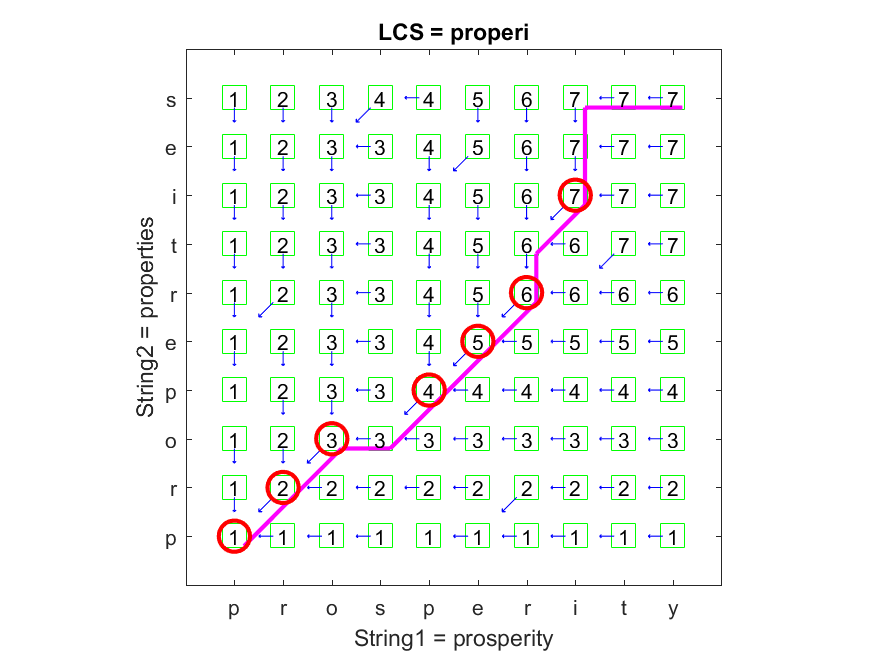8-2 Longest Common Subsequence

[chinese][all]

Given a sequence, we can delete any elements to form a subsequence of the original sequence. For instance, given a string s = "uvwxyz", we can delete v and x to get a subsequence "uwyz". For any given two sequences a and b, the similarity between them can be defined as the length of the longest common subsequence (LCS for short) of these two sequences, which can be computed efficiently by DP.

Let us define the optimum-value function LCS(a, b) as the length of the longest common subsequence between a and b. Then the recursive formula for LCS can be defined as follows:

1. LCS(ax, by) = LCS(a, b)+1 if x = y.
2. LCS(ax, by) = max(LCS(ax, b), LCS(a, by)) if x ≠ y.

The boundary conditions are LCS(a, []) = 0, LCS([], b) = 0.

The following example demonstrates a typical result of LCS of strings "prosperity" and "properties":

Example 1: lcs01.mstr1 = 'prosperity'; str2 = 'properties'; plotOpt = 1; [lcscount, lcsPath, lcsStr, lcsTable] = lcs(str1, str2, plotOpt);As shown in the plot, the circle positions indicate common characters.

It should be noted that the identified sequence is not unique for the above example. The identified sequence is "properi" in the above example, but one can verify that "perpert" is also equally good. In other words, a vanilla DP can only find the one of the best solutions. If you want to find all of the best, you need to modify the back tracking procedure. Moreover, if you want to find the top-n solutions, then you need to invoke the "n-best" version of DP which is a lot more complicated.

Data Clustering and Pattern Recognition (資料分群與樣式辨認)